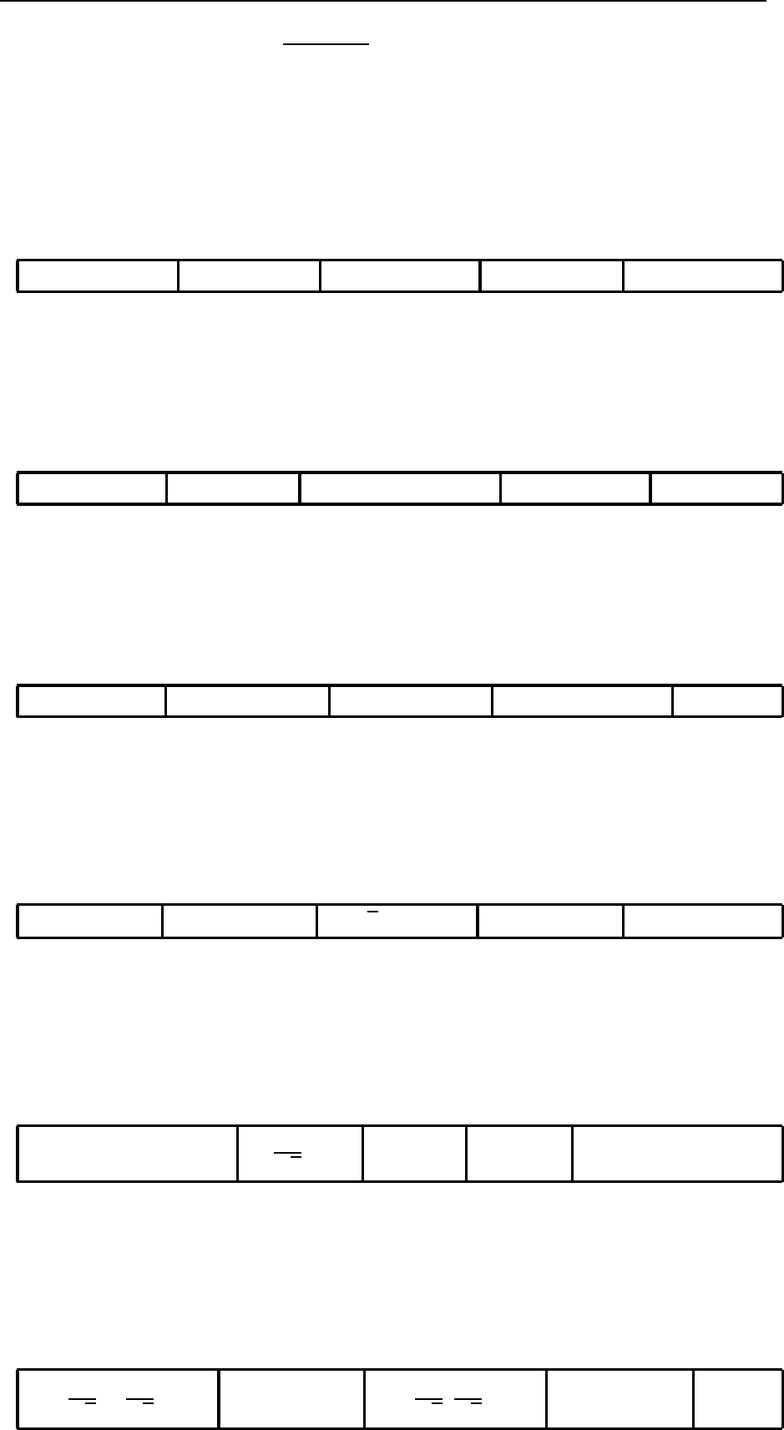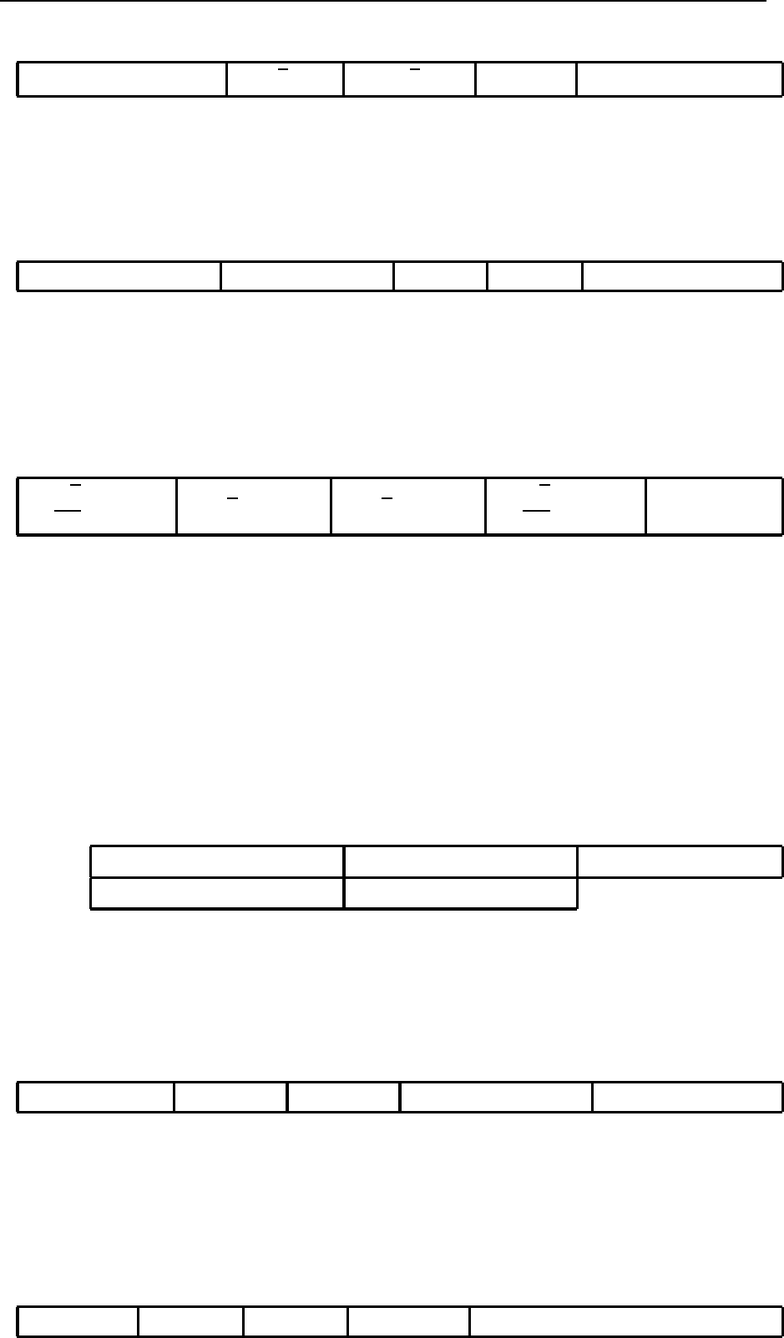Study Guides (380,000)
CA (150,000)
Western (10,000)
MATH (100)
hi (6)
Midterm

# 1229test1 questions.pdf

Department
Mathematics
Course Code
MATH 1229A/B
Professor
hi
Study Guide
Midterm

This preview shows pages 1-2. to view the full 7 pages of the document.Thursday, October 6, 2011
Page 1 CODE 111 Mathematics 1229A
Test 1
PART A (18 marks)
INDICATED ON THE SCANTRON SHEET. YOU SHOULD ALSO CIRCLE
Use the following for questions 1, 2 and 3.
Let u= (1,0,2) and v= (0,1,3).
1.1
mark Find 3u+ 2v.
A: (2,3,5) B: (2,1) C: (3,2,0) D: (3,2) E: (1,2,0)
2.1
mark Find uv.
A:6B: 6 C: (0,0,6) D:4E: 0
3.1
mark Find u×v.
A: (2,3,1) B: (2,3,1) C: (0,0,6) D: (2,3,1) E:6
4.1
mark Find the magnitude (length) of u= 4i3j.
A: 5 B: 25 C:7D: 7 E:±5
5.1
mark Find all values of csuch that the vector u= (c, 1,c, 0) is a unit vector.
A: any real value B:1
2
C: 1 D: 0 E: no real values
6.1
mark Find the unit vector that has the same direction as u= (1,0,1).
A:1
2,0,1
2B: (1,0,1) C:1
2,1
2D: (0,1,0) E:i

Only pages 1-2 are available for preview. Some parts have been intentionally blurred.Mathematics 1229A
Test 1 CODE 111 Thursday, October 6, 2011
Page 2
7.1
mark Find all values of ksuch that the vectors u= (1, k) and v= (2, k) are orthogonal.
A: any real value B:2C:2D: 0 E: no real values
8.1
mark Find all values of asuch that the vectors u= (1,0, a) and v= (a, 0,1) are collinear.
A: any real value B: 1 and 1C: 1 D: 0 E: no real values
9.1
mark Find the area of the triangle which has one vertex at the origin and has the other two
vertices at the points A(1,1,1) and B(1,0,1).
A:6
2B:2C:6D:2
2E: 1
10.1
mark Let u,vand wbe vectors in R3and let cbe a scalar. Which of the following operations
are deﬁned?
(i)c+u(ii)cu(iii)u×(vw)
(iv) (u×v)w(v)u(vw)
A: (ii), (iii) and (iv) only B: (i), (ii) and (v) only C: (ii) and (iv) only
D: (ii), (iv) and (v) only E: All of them.
11.1
mark Find a standard form equation of the plane through the point P(1,0,0) with normal vector
n= (1,2,1).
A:y2z= 0 B:x= 1 C:x= 0 D:x+ 2y+z= 0 E:x+ 2y+z= 1
12.1
mark For what value of tdoes the line x(t) = (1,1,1) + t(0,1,0) yield the point P(1,1,1)?
A:2B: 0 C: 2 D:1E:Pis not on the given line